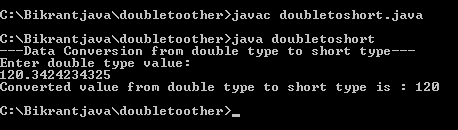# Conversion from double to short

In this tutorial we will learn how to convert a double type value to short type value.

In this tutorial we will learn how to convert a double type value to short type value.

## Conversion from double to short:

In this tutorial we will learn how to convert a double type value to short type value.

## Description:

This program will take a double value from console and provide the conversion to short type. The line double mydouble = Double.parseDouble(buffreader.readLine()); reads the double type value from console. The line short myshort = (short)(mydouble); converts the mydouble double type value to myshort short type data.

## Code:

```import java.io.*;

class doubletoshort {
public static void main(String[] args) {
try {
// Reads the value from console
System.out.println("---Data Conversion from double type to short type---");
System.out.println("Enter double type value: ");
// Convert double type data to short type
short myshort = (short)(mydouble);
System.out.println("Converted value from double type to short type is : " + myshort);
}
catch (Exception e) {
System.out.println(" Error " + e);
}
}
}
```

## Output: